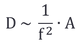top of pagePiezo Accelerometer Tutorial

What is Vibration?

# Basics of Vibration 2

## ​Relation between Acceleration, Velocity and Displacement

So far we have set all amplitudes to "one unit" i.e. we have chosen the scale to plot the curves in a way that they appeared uniform with the same amplitude. This made it easier to show the basic characteristics of sinusoidal vibration and particularly the phase shift between a, v and d.

However the amplitudes of acceleration, velocity and displacement are always in a determined relation to each other. This relation is given by the frequency.

In the following we want to explore this law  in more details.

In a harmonic vibration we can choose one amplitude (for example the acceleration) and the frequency. With this set the other amplitudes (velocity and displacement) will be in a fixed relation.

With constant acceleration and increasing frequency

the velocity decreases proportionally with the inverse frequency:

The displacement decreases with the

inverse frequency squared:For low frequencies the values for v and d become very large and for high frequencies very small.

For this reason we normally use logarithmic scales for the frequency and the amplitude.

A logarithmic scale is non-linear. The values increase always a distinct factor from division to division. For example a factor 10 like on the sample on the right.

This allows showing the values over several decades.The velocity amplitude V decreases -1 decade per decade of the frequency

and the displacement amplitude D decreases -2 decades per decade of the frequency.

Relation between A / V / D

## ​Dimensions of Acceleration, Velocity and Displacement

In the chapter about linear acceleration we have seen the dimensions of the vibration parameters

which are:

displacement :   meters (m) or milli-meters (mm)

velocity :            meters per second (m/s) or milli-meters per second (mm/s)

acceleration :     meters per second per second (m/s²)

These are also the correct dimensions to use for the vibration terms in the SI-system

However in wide parts of the industry particularly in aeronautics we use also an English system with the following units:

displacement :   inch (in) or mils (in/1000)

velocity :            inch/second (ips)

acceleration :     g ( = acceleration of gravity)

1g  =  9.81 m/s²